Next: Linear computational complexity Up: Building Blocks for Hierarchical Previous: Introduction

# Ensemble Learning

This section gives a brief overview of ensemble learning with emphasis on solutions yielding linear computational complexity. Thorough introductions to ensemble learning can be found for instance in [13,14].

Ensemble learning is a method for approximating posterior probability distributions. It enables to choose a posterior approximation ranging from point estimates to exact posterior. The misfit of the approximation is measured by the Kullback-Leibler divergence between the posterior and its approximation. Let us denote the observed variables by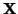, the latent variables (parameters) of the model by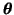and the approximation of the true posterior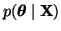by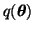. The cost function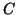used in ensemble learning is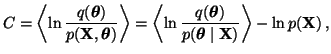(1)

where the operator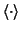denotes an expectation over the distribution. Note that for practical reasons the cost function equals the Kullback-Leibler divergence only up to a constant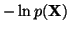. This means that the cost function can be turned into a lower bound of the model evidence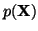which can then be used for learning the model structure.

SubsectionsNext: Linear computational complexity Up: Building Blocks for Hierarchical Previous: Introduction
Harri Valpola 2001-10-01